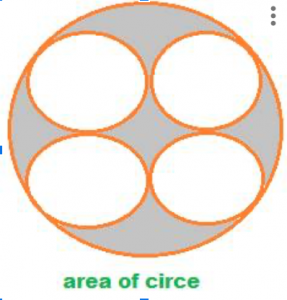Consider the circle below with a radius ‘r’. Area of a circle, in lay terms, is the amount of region enclosed by the green curve going round the point C. Area of a circle, technically, is Π times the radius squared. i.e. Area of a circle = Π × r2. Area of a circle, if diameter length is d, is Π × (d2/4). {since radius is half of diameter} Write either 22/7 or approximately 3.14 for Π. Let us solve a few questions on area of a circle, when different parameters are given.

## Example 1:

Find the area of a circle whose radius is 7 cms.

Substitute 7 in radius, r in the area of a circle, Π × r2. So, area of circle is Π × 72 = (22/7) × 497 = 22 × 7 = 154. Expressed along with units, the area of circle is 154 sq. cms.

## Example 2

Find the area of a circle whose circumference is 88 cms.